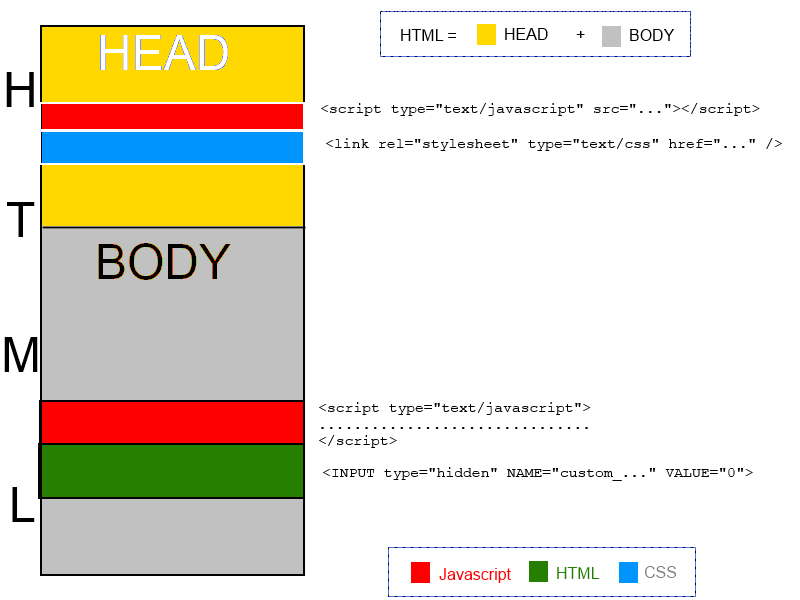# Custom question type : general concepts

## Custom question type : general conceptsAny new question type in QuizFaber must be defined using three different programming languages:

1. HTML : it defines the model of a question type
2. Javascript : it is the "engine" that manage the events that happens on HTML page and, when the "OK" button is pressed, decide if the question was answered correctly or not.
3. CSS : it defines the external style, how the model is rendered into HTML page, the graphic aspect that you see.

As in any HTML page, there are two top-level sections, called "HEAD" (the upper side) and "BODY" (the lower side).

In the HEAD section, a new question type can define (if there are any) one or more external files, that are:

• Javascript files (with .JS file extension)
• CSS files (with .CSS file extension)

The files are copied into quiz file (.XQZ). So, If you wish modify them, after the changes in the original file, you should re-import that file into QuizFaber.

In the BODY section, a new question type must define (it is mandatory) two sections:

1. A Javascript code (tipically a function)
2. The HTML code of the component that defines the new question. For example, if it is a "numerical answer", it define a HTML INPUT tag, with TEXT type that represent the numeric box in which the student writes the answer.

Moreover, it should be defined (if there are) two more things, that are referred to section "BODY":

1. A list of multimedia objects, for example, in a "jigsaw question type", the file that defines the image that have to divided into pieces and re-assembled.
2. A list of parameters.

A parameter has a name, a type (integer number, real number, text alias "string" in programming languages), a default value and a true or false value that says if the parameter value should be encrypted into HTML page or not.
In every place of your Javascript and HTML code (only for BODY section), you can use a parameter instead of value (numeric or text) that parametrizes the question type, so you can have in your quiz different questions of same type, and with different parameter values.

In every question type, there are four already defined parameters that are:

• %NUM% : the question number.
• %NAME% : the name of the question type.
• %MEDIA_DIR% : the relative path for the images associated with the custom question, to be used for example for the SRC attribute of the html <IMG> tag.
• %CALL_EVALUATION% : in the cas of is not required an explicit confirmation of the selected answer by the user, it is the final Javascript function that must be called from the code when the logic determines that the user has definitively chosen his answer.

The rule is that, into the code, a parameter name is preceded by a percent (%) sign and it is followed by another percent sign.

There is an important conjunction point between the code written for the new question type and the quiz engine. It is the following HTML line:

<INPUT type="hidden" NAME="custom_%NAME%_%NUM%" VALUE="0">

How you can see, here appears the default parameters, NAME and NUM. For example for a new question type called "jigsaw", used into the question number 8, the line will be translated in:

<INPUT type="hidden" NAME="custom_jigsaw_8" VALUE="0">

The "VALUE" attribute of the HTML object called "custom_jigsaw_8" says to the quiz engine if the answer to question was correct (VALUE set to 1) or was wrong (VALUE set to 0).

Thus, in Javascript code, "VALUE" attribute is set in the following way:

• for correct answer : \$('#custom_%NAME%_%NUM%').val(1);